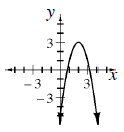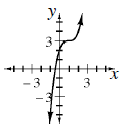### Home > CCA2 > Chapter Ch7 > Lesson 7.1.4 > Problem7-58

7-58.

Sketch a graph of each equation below.

1. $y = −2\left(x − 2\right)^{2} + 3$1. $y = \left(x − 1\right)^{3} + 3$